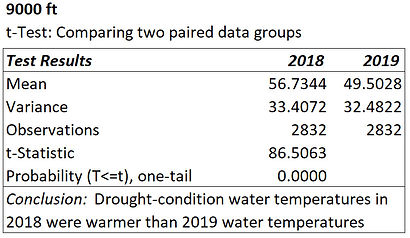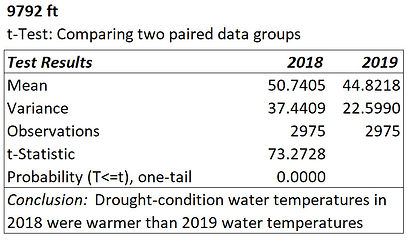# t-Test, temperatures

## A. Background

1. July-August 2018 mean flow in the main stem was 22 cfs, the second lowest since 1951, when records-keeping began.

2. The following year, in contrast, mean July-August flow was 226 cfs, an order of magnitude greater than in 2018.

3. The hydrological measurements are from the main-stem Rico station, 8422 ft, USGS location 09165000.

4. Historical mean flow from 1951 to 2020 during the July-August period was 122 cfs; daily median flows, 45-160 cfs.

5. Clearly, drought conditions prevailed when July-August water temperature were measured in 2018 , but not in 2019.

## B. Question​

1. Mean water temperatures were 5 F higher in 2018 than in 2019 in the main stem at 7000 ft; 7 F higher at 9000 ft.

2. Were they differences of separate temperature conditions, or simply part of temperature variation at the sites?

3. Is there a sound statistical basis for concluding that main-stem drought-condition temperatures were warmer?

4. Would that appear to extend to tributaries, considering, as examples, a large and small one?C. Application

1. July-August 2018 water temperatures from 7000 and 9000 ft in the main stem were compared with July-August 2019.

2. Also, they were compared from 9502 and 9792 ft at Slate, a large tributary, and 8510 ft at Tenderfoot, a small one.

3. Slate, outfall at 9502 ft, is the 7th largest-flow tributary and the 6th largest in drainage area and maximum elevation.

4. Tenderfoot, outfall at 8222 ft, is the 37th largest of the study's 42 streams, much smaller than Slate.

D. Assessment

1. For a statistically sound comparison, a paired t-test was used, which assesses data means.

2. Paired, in this case, referred to water temperature measurements being repeated in location, frequency, and duration.

3. Additionally, the databases generated met the requirements of being continuous, independent observations.

4. Also, the databases were large, 2932 measurements at the main stem sites and 2975 at the tributary sites.

5. Being large, treating the data as normally distributed was appropriate, in consideration of the central limit theorem.

6. That is, using means to represent the temperature data would be correct.

E. Findings

1. t-test results from comparing the water temperature means are shown in the tables below.

2. The t-statistics for the main stem were high: 52 for comparison of 2018 and 2019 data at 7000 ft, and 87 at 9000 ft.

3. For both elevations, the P-value was zero, which is below a regularly used significance level of 0.05, or even 0.001.

4. "One-tail" refers to assessing a change in temperature in one direction, an increase or a decrease, but not both.

5. The t-statistics for Slate also were high: 87 for comparison of 2018 and 2019 data at 9502 ft, and 73 at 9792 ft.

6. For both Slate elevations, the P-value was zero, also below routinely considered significance levels of 0.05 or 0.001.

7. The t-statistic for Tenderfoot was high: 54 for comparison of 2018 and 2019 data.

8. The P-value was zero for Tenderfoot, again, below commonly applied significance levels of 0.05 or 0.001.

9. The larger the t-statistic and the smaller the P-value, the greater is the statistical soundness of temperature difference.

10. Expressed another way, there is reason to be >99.9% confident in the assessment findings.

F. Conclusions

1. Based on these outcomes, the null hypothesis of no difference in 2018 and 2019 temperatures should be rejected.

2. It should be concluded that, as compared with 2019, the 2018 temperatures measured during drought were warmer.

3. That is, the data show clearly that they represent different temperature conditions in 2018 and 2019.

G. Criteria

1. Important, in particular, was how those temperature conditions compared with CO chronic and acute criteria.

2. The maximum weekly average temperatures (MWAT) at 7000 ft were above the chronic criterion both years.

3. The MWAT at 9000 ft were above that criterion neither year.

4. The MWAT at 7000 was 6 F higher in 2018 and than in 2019; at 9000 ft, it was 8 F higher

5. The maximum two-hour average temperatures (MTAT) at 7000 ft were above the acute criterion both years.

6. The MTAT was above the acute criterion at 9000 ft only in 2018, the drought year; see WAT-TAT summary.

7. The MTAT at 7000 ft was 7 F higher in 2018 and at 9000 ft, 9 F higher, both as compared with 2019.

##### t-Test of main-stem drought & non-drought water temperature differences##### t-Test of Slate drought & non-drought water temperature differences##### t-Test of Tenderfoot drought & non-drought water temperature differencesSources

1. Hydrologic information from https://nwis.waterdata.usgs.gov/nwis

2. t-Test information from application of Microsoft Excel